Mobile QR Code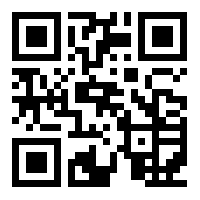1. (Research Scholar, Reva University, Asst. Professor, Dept. of ECE, Govt. Engineering College, Devagiri, averi-581110 yashvanth3@gmail.com )
2. (Dept. of ECE, NMIT, Bangalore slpinjare@gmail.com)
3. (Professor, Cambridge Institute of Technology, Bangalore cyrilyahoo@gmail.com)

Distributive arithmetic algorithm, Memory efficient, Wavelet transform, Image processing, FPGA

## 1. Introduction

Wavelet-based image processing algorithms provide edge information in a given image localized in sub-bands and at different resolutions, along with the intensity component of the image. The Discrete Wavelet Transform (DWT) of an image generates sub-bands that capture intensity and directional features that are localized. Directional orientations in directions other than 0$^{\mathrm{o}}$, 90$^{\mathrm{o}}$and 45$^{\mathrm{o}}$ are captured in Dual Tree Complex Wavelet Transforms (DTCWTs). Shift invariant and additional directional orientation are the major advantages of DTCWT over DWT. The number of filters is twice as high in DTCWT than DWT, and the computation complexity is 2$^{\mathrm{n}}$:1.

The use of DTCWT over DWT for image processing applications is widely reported in the literature [1-3]. Hardware implementation methods for DTCWT-based image processing provide a choice for real time applications. The filter structure in DTCWT is similar to DWT, and most of the hardware architectures reported in the literature for DTCWT are extensions of DWT architecture.

It is required to develop customized hardware architectures for DTCWT. Hardware models based on the Systolic Array (SA) algorithm and Distributive Arithmetic (DA) algorithm are widely used to develop fast architectures for DWT computation. Mohanty and Meher reported multiplier-less structures considering 9/7 filters and designed a DA structure with serial and parallel data-processing modules . Mahajan and Mohanty designed arithmetic modules for a DA-based DWT structure to minimize the critical path and to improve processing speed . Mohanty and Meher developed a multi-level decomposition structure for computing DWT sub-bands for image processing applications by optimizing memory requirements and improving operating speed .

Aziz et al. developed a DA-based DWT architecture based on 5/3 filters . Similarly, Gardezi et al. used a canonical sign digit number system to reduce the number of arithmetic operations for 2D DWT computation . Naik et al. developed a DWT IP that can be reconfigured and implemented it on Xilinx FPGA, demonstrating a delay of 11.577 ns and total power dissipation of less than 23.8 mW . Anirban and Ayan developed a DA-based architecture for DWT, improving processing speed and memory efficient structure for 1D and 2D computation . The DWT architectures reported in the literature are either for a 9/7 filter or 5/3 filter. In DTCWT computation, 10-tap filter size is used, and it is required to redesign the architectures that have been developed for 9/7 and 5/3 filter structures.

Poornima et al. extensively developed high-speed architectures based on systolic array logic for computing DTCWT and reported its implementation on an FPGA, limiting power dissipation to less than 10 mW . Divakara et al. reported a hybrid DA structure for 2D DTCWT computation . The methods presented in their work combine SA and DA logic to develop a DTCWT architecture and were implemented on an FPGA, demonstrating optimization in area, power, and speed performance .

In DTCWT, as there are four filters in each stage, and each of the filters is 10-tap, there are redundancies that need to be considered, and it is required to compute the filter outputs by combining all operations and eliminating redundancies. In this work, new methods were developed to identify redundancies among the filters and processing elements. Data movement and reuse of arithmetic operations were considered in design of novel methods for DTCWT computation. The developed method was implemented on an FPGA, and its performance was compared with other methods.

## 2. Related Work

The most popular DTCWT structure is based on a Kingsbury 10-tap filter. The 2D DTCWT structure is presented in Fig. 1. The input X(n1, n2) represents 2D data and is processed by two stages of filters. In the first stage, there are four filters: L$^{1}$a, H$^{1}$a, L$^{1}$b, and H$^{1}$b, which are low pas real, high pass real, low pass imaginary, and high pass imaginary filters, respectively. The input X is processed along the rows to generate the first-stage filter outputs, which are further processed by the second-stage filter banks.

In the second stage, the processing is carried out along the columns of the first-stage output. The filter coefficients for the second stage are represented as L$^{2}$a, H$^{2}$a, L$^{2}$b, and H$^{2}$b. The second-stage filter generates eight complex sub-bands represented as XLL$_{1}$, XLL$_{2}$, XLH$_{1}$, XLH$_{2}$, XHL$_{1}$, XHL$_{2}$, XHH$_{1}$, and XHH$_{2}$. Considering real sub-bands, there are 16 sub-bands, of which 4 are low pass and 12 are high pass. The filter coefficients for first stage are presented in Table 1.

The number of sub-bands generated by DTCWT is 16, which is 4 times higher than DWT sub-bands. The filter coefficients in the second stage are different from stage-one filter coefficients. Considering 10 filter coefficients per filter, the input data are processed along the rows in the first stage and along the columns in the second stage. If there are N inputs in each of M rows, the total numbers of multiplications and additions to process every row are 10N and 9N, respectively.

Considering M rows with each row having N elements, the total numbers of multiplications and additions are 10MN and 9MN, respectively. For the first stage, as there are four filters, the total numbers of multiplications and additions are 40MN and 36MN, respectively. For the second stage, the total numbers of multiplications and additions are 80MN and 72MN. The total numbers of multiplication and addition operations for level-1 decomposition are 120MN and 108MN, respectively. In addition to arithmetic operations, the intermediate memory elements required are 4NM for the first stage and 16NM for the second stage. The propagation delay is one of the major challenges in DTCWT computation as the processing structure needs to process the entire image.

It is required to reduce both the arithmetic and memory operations to optimize the area and speed. The filter coefficients require 16-bit signed bit representation, and this will further increase the computation complexity of the arithmetic operations. Scaling the filter coefficients by 256, rounding off to the nearest integer, and using 2’s complement signed representation reduces the computation complexity as the number of bits is reduced from 16 bits to 9 bits.

The level-1 sub-bands after decomposition generate 16 sub-bands each of size N/2 x M/2. The low pass sub-band is further decomposed to level-2 and level-3 sub-bands if three-level decomposition is considered. In order to reduce the computation complexity or to reduce the number of arithmetic operations and propagation delay, a DA algorithm was considered, and the algorithm was modified for DTCWT computation.

##### Fig. 1. DTCWT level-1 decomposition structure for 2D data.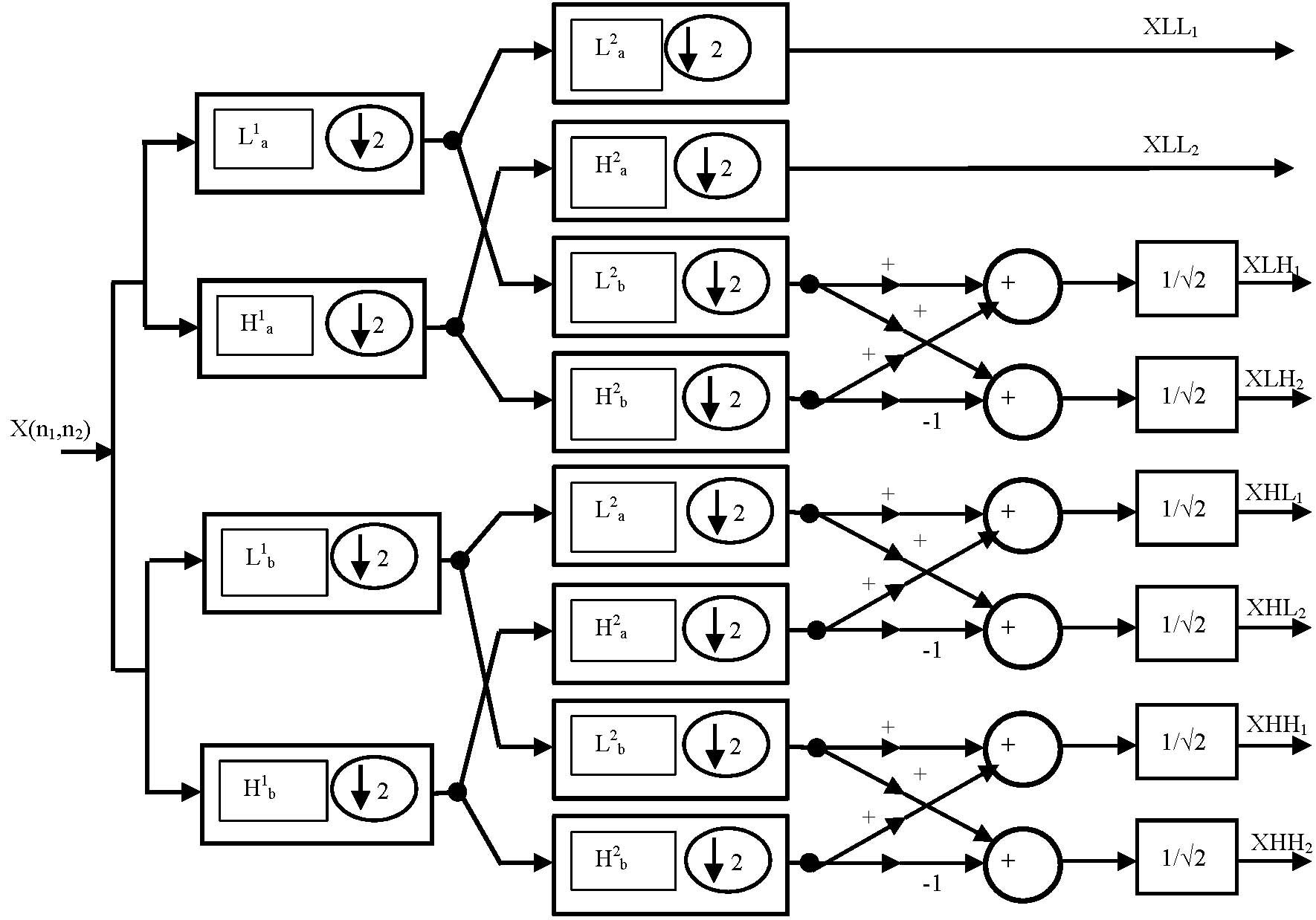##### Table 1. DTCWT filter coefficients for level-1 decomposition.
 L1a H1a 0 0 0 0 -0.08838 -23 -0.01122 -3 0.08838 23 0.01122 3 0.69587 178 0.08838 23 0.69587 178 0.08838 23 0.08838 23 -0.69587 -178 -0.08838 -23 0.69587 178 0.01122 3 -0.08838 -23 0.01122 3 -0.08838 -23 0 0 0 0 L1b H1b 0.01122 3 0 0 0.01122 3 0 0 -0.08838 -23 -0.08838 -23 0.08838 23 -0.08838 -23 0.69587 178 0.69587 178 0.69587 178 -0.69587 -178 0.08838 23 0.08838 23 -0.08838 -23 0.08838 23 0 0 0.01122 3 0 0 -0.01122 -3

## 3. DA Architecture for DTCWT

In order to determine the primary path switching criteria for each MT, we take into account two metrics, including the historical relative difference of RTT against the estimated movement speed of MT. Both metrics are calculated only when MT is in dual-homing mode as it is located in the overlapping state among different networks, as shown in Fig. 1. In order to simplify our description, we assume that each MT has only one alternative path since the proposed scheme can be applied in a straight forward manner to the multi-alternative paths environment.

In the DA algorithm, the input data is represented using 2’s complement signed numbers. The filter coefficients are grouped together considering the binary weighting of input data bits. As the filter coefficients are constant, grouping of coefficients based on binary weighting of input data bits leads to partial products that are predefined based on input bit combinations. In the proposed DA algorithm, the number of partial products is reduced considering the redundancies in DTCWT filters. The proposed DA algorithm is presented in this section. A generic convolution operation for FIR filter is mathematically represented as Eq. (1),

##### (1)
$Y=\sum _{k=1}^{k}A_{K}X_{K~ ~ ~ ~ ~ ~ ~ }$
Y is the filter output, X is the input, and A is the filter coefficient. The input X is represented in 2’s complement in Eq. (2):
##### (2)
$X_{K}=-b_{k0}+~ \sum _{n=1}^{N-1}b_{kn}2^{n-1}$

$b_{k0}$ is the sign bit, and b$_{\mathrm{kn}}$ represents the binary bits. Substituting Eq. (2) in Eq. (1), the FIR filter output is given as Eq. (3):

##### (3)
$Y=\sum _{K=1}^{K}A_{k}\left[-b_{k0}+\sum _{N=1}^{N-1}b_{kn}2^{-n}\right]$

The FIR output in Eq. (3) is rearranged by expanding the terms, and by reorganizing, the summation Eq. (4) is obtained.

##### (4)
$~ Y=\sum _{K=1}^{K}\left(b_{k0}A_{k}\right)+\sum _{k=1}^{k}\sum _{n=1}^{N-1}\left(A_{K}.b_{kn}\right)2^{-n~ }$

In Eq. (4), the first term is the sign bit, and the second term is the partial product term. For different combinations of n, the weighted binary bits b$_{\mathrm{kn}}$ are multiplied by the fixed coefficients A$_{\mathrm{k}}$, and the partial products are pre-computed. These pre-computed partial products are stored in the memory.

The memory elements are accessed by considering the input data as the address and each of the partial products is accumulated to generate the filter output. In DTCWT, there are four filters in the first stage. Considering Eq. (4), the output for the two filters is expressed in Eq. (5). The suffix x in Eq. (5) is either a or b, denoting real and imaginary terms and from this, an expression for all four filters can be found.

##### (5a)
$Y_{Lx}=-\sum _{K=1}^{10}b_{k0}L_{xk}+\sum _{k=1}^{10}\left[\sum _{n=1}^{N-1}(b_{kn}L_{xk})2^{-n}\right]$
##### (5b)
$Y_{Hx}=-\sum _{K=1}^{10}b_{k0}H_{xk}+\sum _{k=1}^{10}\left[\sum _{n=1}^{N-1}(b_{kn}H_{xk})2^{-n}\right]$

As there are 10 filter coefficients, the expression is suitable set for computing DTCWT filter outputs. As there are 10 filter coefficients, there will be 2$^{10}$ possible partial products. As there are four filters, the total number of memory units required will be 4 memory units with size of 1024 x 10 (40960) bits each. In order to reduce the number of partial products and to increase processing speed, a modified algorithm was developed. Considering Eq. (5a), by splitting the second in Eq. (5a) into two equal terms as in Eq. (6), the number of partial products is reduced and can be reused.

##### (6)
$Y_{La}=\sum _{K=0}^{4}\sum _{n=1}^{N-1}\left[\left(L_{ak.}b_{kn}\right)2^{-n}\right]+ \\ \sum _{K=5}^{9}\sum _{n=1}^{N-1}\left[\left(L_{ak.}b_{kn}\right)2^{-n}\right]$

In the reorganized DA algorithm expression, the first term and second term have 5 filter coefficients, and hence, the number of partial products for each term is 2$^{5}$. The total number of storage memory bits required is 640 bits. By splitting the expression, the total number of bits to store partial product is reduced by 98.43%.

Table 2 presents the partial products for four filters based on the modified DA algorithm expression in Eq. (6). The block diagram for the modified DA algorithm is presented in Fig. 2. The input is stored in a PISO register of depth 10. Once the data is loaded into the PISO, the LSBs from each of the 10 registers are read out to form the address for the memory unit. As the memory unit is split into two sections, the LSBs from the top five registers are used as an address for memory unit 1, and the LSBs from the bottom 5 registers are used as an address for memory unit 2.

Each partial product read out from look up tables (LUTs) is accumulated in the accumulator section, and the final output is generated at the output of the summer. As the input data width is 9, the partial products are read out 9 times for different combinations of address bits, and the accumulator performs an operation 9 times. In the 10$^{\mathrm{th}}$ clock, the final output is generated at the output of the summer. The latency of the modified DA structure is 20 clocks (10 clocks for data loading into PISO and 10 clocks for data reading and accumulation).

It was observed that the contents of YLa$_{\mathrm{LUT1}}$, YHa$_{\mathrm{LUT1}}$, YLb$_{\mathrm{LUT1}}$, and YHb$_{\mathrm{LUT1}}$ from memory locations 10000 to 11111 were 178, 23, 23, and -178 higher than memory contents from locations 00000 to 01111. It was also observed that the memory contents of YLa$_{\mathrm{LUT2}}$, YHa$_{\mathrm{LUT2}}$, YLb$_{\mathrm{LUT2}}$, and YHb$_{\mathrm{LUT2}}$from memory locations 00000 to 01111 were similar to the contents in memory locations 10000 to 11111. Based on these observations, the reduced memory DA algorithm was designed.

##### Fig. 2. Modified DA structure for YLa filter.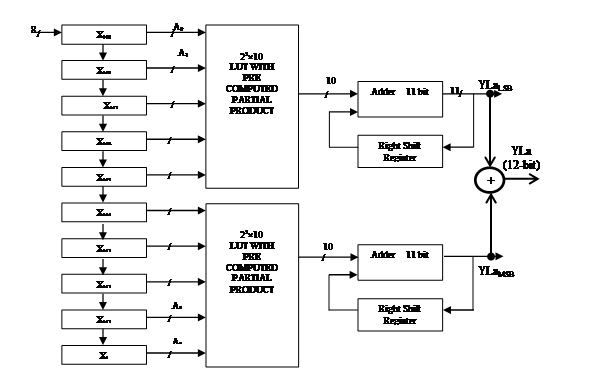##### Table 2. LUT contents of DTCWT filter.
 A4/9A3/8A2/7A1/6A0/5 YLaLUT1 YLaLUT2 YHaLUT1 YHaLUT2 YLbLUT1 YLbLUT2 YHbLUT1 YHbLUT2 00000 0 0 0 0 0 0 0 0 00001 0 23 0 -178 0 0 0 23 00010 -23 -23 -3 178 3 178 -23 23 00011 -23 0 -3 0 3 178 -23 46 00100 23 3 3 -23 3 23 -23 3 00101 23 26 3 -201 3 210 -23 26 00110 0 -20 0 155 6 210 46 26 00111 0 3 0 -23 6 379 -46 49 01000 178 3 23 -23 23 -23 178 -3 01001 178 26 23 -201 23 -23 178 20 01010 155 -20 20 155 26 155 155 20 01011 155 3 20 -23 26 155 155 43 01100 201 6 26 -46 26 0 155 0 01101 201 29 26 -224 26 178 155 23 01110 178 -17 20 132 29 178 132 23 01111 178 6 20 -46 29 356 132 46 10000 178 0 23 0 23 0 -178 0 10001 178 23 23 -178 23 0 -178 23 10010 155 -23 20 178 26 178 -201 23 10011 155 0 20 0 26 178 -201 46 10100 201 3 26 -23 26 23 -201 3 10101 201 26 26 -201 26 210 -201 26 10110 178 -20 20 155 29 210 -224 26 10111 178 3 20 -23 29 379 -224 49 11000 356 3 46 -23 46 -23 0 -3 11001 356 26 46 -201 46 -23 0 20 11010 333 -20 43 155 49 155 -23 20 11011 333 3 43 -23 49 155 -23 43 11100 379 6 49 -46 49 0 -23 0 11101 379 29 49 -224 49 178 -23 23 11110 356 -17 43 132 52 178 -46 23 11111 356 6 43 -46 52 356 -46 46

### 3.1 Memory Efficient DA Architecture

Table 3 presents the LUT content for four filters considering only term 1 of the expression presented in Eq. (6). The LUT contents provided are regrouped into two components considering the MSB address A4. If address A4 is 0, the contents of LUT are YLa$_{\mathrm{LUT1}}$, YHa$_{\mathrm{LUT1}}$, YLb$_{\mathrm{LUT1,}}$and YHa$_{\mathrm{bUT1}}$. If the address A4 is 1, the contents of LUT are 178 + YLa$_{\mathrm{LUT1}}$, 23 + YHa$_{\mathrm{LUT1}}$, 23 + YLb$_{\mathrm{LUT1,}}$and 178 + YHa$_{\mathrm{bUT1}}$. The address bits A$_{3}$, A$_{2}$, A$_{1}$, and A$_{0}$ are used to access the LUT contents, and the LUT depth is 16.

The DA structure for Term 1 of the YLa filter is presented in Fig. 3. The five input registers store the input data, and the LSBs of these registers are connected to the LUT address bits. The LSB of the top register is considered as address bit A4, which is connected to the multiplexer select line. At every clock, the LSBs of all four registers are used to read out the LUT content. The output of the LUT is accumulated according to the combinations of address bits, and the accumulated content is sent to the output summer.

The summer circuit performs addition of the accumulated output along with a constant number that is read from the output of 2:1 multiplexer. For the YLa filter, the constants are 0 and 178, which are used in the summer circuit to generate the final output and are stored in output register Reg1. The DA structure for YHa term 1 filter is presented in Fig. 4, and the constants are 0 and 23. Similarly, the DA structure for YLb and YHb filters were designed. The DA structure for term 2 for all four filters was deigned considering the LUT contents presented in Table 4. For the address bits A$_{3}$, A$_{2}$, A$_{1}$, and A$_{0}$, the LUT contents remain the same for both possible conditions of MSB address bit A$_{4}$. Considering the LUT contents for all four filters indicated in Table 5, the LUT size will be 16. Upon observation of LUT data, the partial products of the LUT for address bits 0000 to 0111 and 1000 to 1111 are repeated. The contents of Tables 4 and 5 are arrived considering optimum number of entries required for computing DTCWT partial products and for efficient implementation of the architecture on FPGA platform.

Considering the redundancy in the LUT content, further modification was carried out, and the contents of LUT were reduced as presented in Table 5. The address bits A$_{2}$, A$_{1}$, and A$_{0}$ are used to access the LUT contents, and the address bits A$_{4}$ and A$_{3}$ are used to enable a constant number to be added with the accumulated output at the summer circuit. The LUT contents for YLa and YHa filter term 2 are shown in Table 5. Similarly, the LUT content for YLb and YHb can be identified. Fig. 5 presents the reduced memory DA structure. The top two registers from the address bits A4 and A3 are not connected to the LUT address bits. The bottom three registers’ LSBs form the address of the LUT.

The depth of the LUT is 8, and the LUT content is read out every clock and accumulated at the accumulator module. The accumulated data is given to the summer to add the corresponding constant depending upon the status of address bit A3. The summer output is stored in the register Reg3. The final output of the filter is generated by summing the output of Reg1 and Reg3. Direct implementation of the DTCWT filter using DA algorithm will require 10 input registers, a LUT of size 1024 x 10, and an 11-bit accumulator. The total propagation delay will be T$_{\mathrm{LUT}}$ + T$_{\mathrm{ACC}}$, which represents the delay of LUT data readout and delay in the accumulator.

With four filters in the first stage, the total number of sub-modules required is presented in Table 6 and compared with the proposed optimized structure. The advantages of the proposed method are in terms of memory size of LUTs. The proposed method requires two LUTs per filter of size 16 x 10 and 8 x 10 (10 is the bit width of LUT contents). Compared with the direct method of implementation, the savings in memory size are 97.65% per filter. The number of adders and accumulators are increased by 3 and 1 compared with direct implementation, respectively. The critical path is increased by 2T$_{\mathrm{ADD}}$, and latency and throughput are increased by 2 clock cycles.

##### Fig. 3. Memory efficient DE structure for YLa term 1 filter.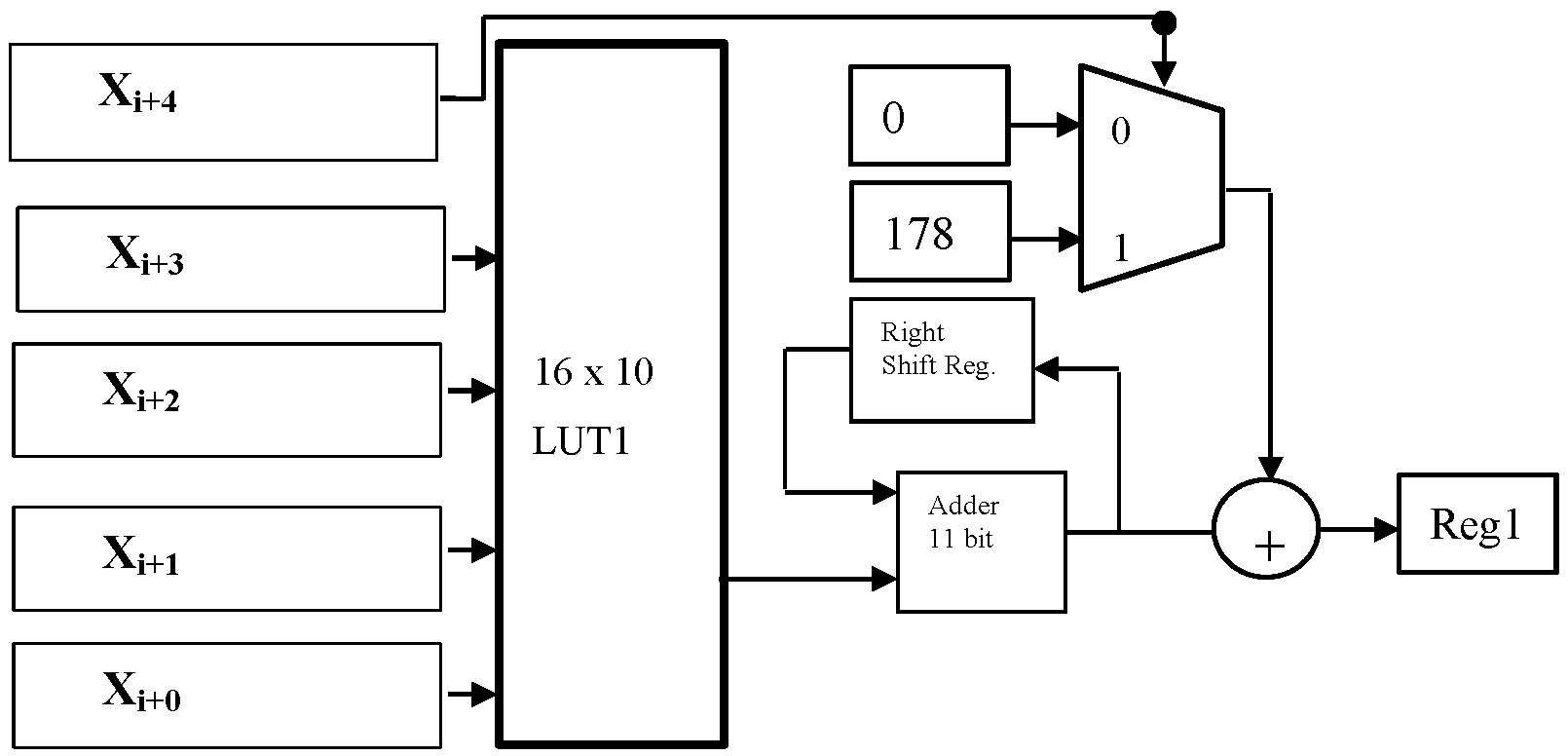##### Fig. 4. Memory efficient DA structure for YHa term 1 filter.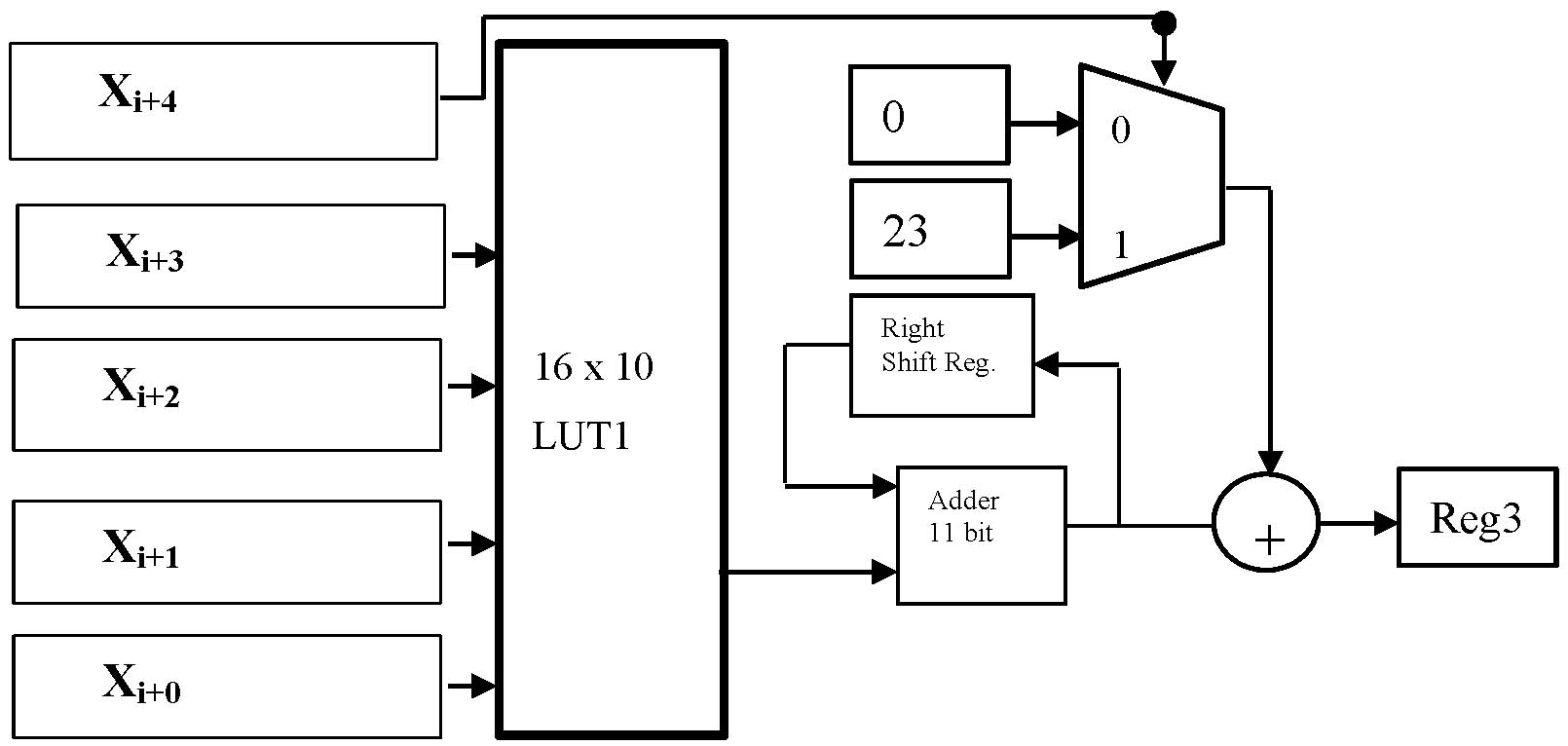##### Fig. 5. Reduced memory DA structure for term 3 filter.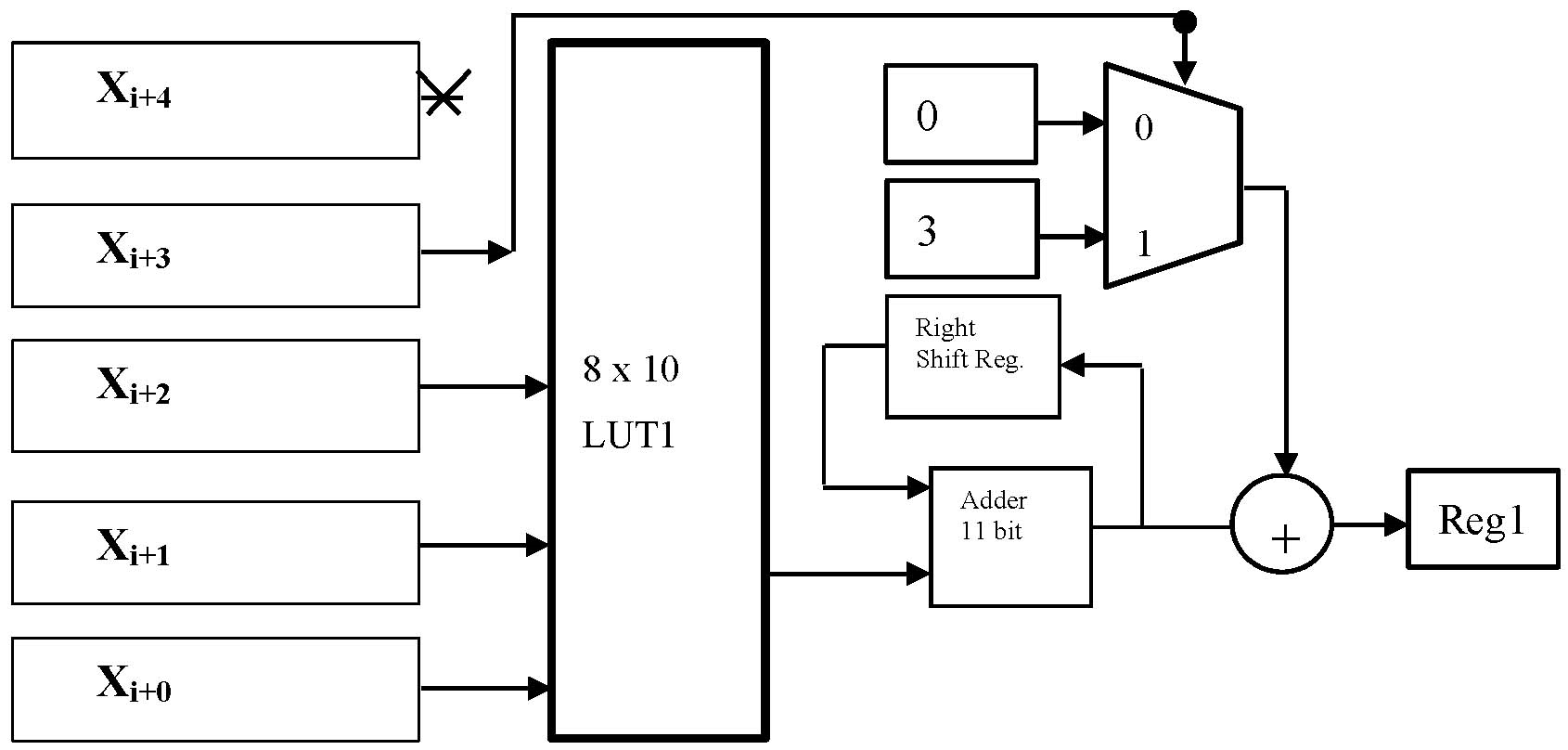##### Table 3. Modified LUT contents for memory efficient DA (Term 1).
 L1a Filter Term 1 H1a Filter Term 1 Address bits A4=0 A4=1 A4=0 A4=1 A3 A2 A1 A0 YLaLUT1 178 + YLaLUT1 YHaLUT1 23 + YHaLUT1 0000 0 0 0 0 0001 0 0 0 0 0010 -23 -23 -3 -3 0011 -23 -23 -3 -3 0100 23 23 3 3 0101 23 23 3 3 0110 0 0 0 0 0111 0 0 0 0 1000 178 178 23 23 1001 178 178 23 23 1010 155 155 20 20 1011 155 155 20 20 1100 201 201 26 26 1101 201 201 26 26 1110 178 178 20 20 1111 178 178 20 20
##### Table 4. Modified LUT contents for memory efficient DA (Term 2).
 Address bits L1a Filter Term 2 H1a Filter Term 2 A4=0 A4=1 A4=0 A4=1 A3 A2 A1 A0 YLaLUT2 YLaLUT2 YHaLUT1 YHaLUT1 0000 0 0 0 0 0001 23 23 -178 -178 0010 -23 -23 178 178 0011 0 0 0 0 0100 3 3 -23 -23 0101 26 26 -201 -201 0110 -20 -20 155 155 0111 3 3 -23 -23 1000 3 3 -23 -23 1001 26 26 -201 -201 1010 -20 -20 155 155 1011 3 3 -23 -23 1100 6 6 -46 -46 1101 29 29 -224 -224 1110 -17 -17 132 132 1111 6 6 -46 -46
##### Table 5. Reduced memory DA contents for term 2 filters.
 Address bits L1a Filter Term 2 L1a Filter Term 2 A4 A3=00 A4 A3=01 A4 A3=10 A4 A3=11 A2 A1 A0 YLaLUT2 3 + YLaLUT2 YHaLUT1 3 + YHaLUT1 000 0 0 0 0 001 23 23 23 23 010 -23 -23 -23 -23 011 0 0 0 0 100 3 3 3 3 101 26 26 26 26 110 -20 -20 -20 -20 111 3 3 3 3
##### Table 6. Comparison of DA methods.
 Implementation method Filter LUT Size No. of adders No. of accumulators Critical path (T) Clock delay Latency Throughput Direct DA YLa 1024 0 1 TLUT +TACC 21 12 YHa 1024 0 1 21 12 YLb 1024 0 1 21 12 YHb 1024 0 1 21 12 Split DA YLa 32 1 2 TLUT +TACC + TADD 22 13 YHa 32 1 2 22 13 YLb 32 1 2 22 13 YHb 32 1 2 22 13 Proposed DA YLa 24 3 2 TLUT +TACC + 2TADD 23 14 YHa 24 3 2 23 14 YLb 24 3 2 23 14 YHb 24 3 2 23 14

## 4. FPGA Implementation

The top level module for DTCWT computation comprises four filters: YLa, YHa, YLb, and YHb. They are represented as two pairs of filter banks denoted as tree a'' and tree b''. The tree a'' filter bank is YLa and YHa, and the tree b'' filter band is YLb and YHa. Each of these filter banks are modeled using Verilog HDL. The behavioral model for term 1 and term 2 of each of the filters was developed and verified for its functionality. The verified HDL model was integrated into a higher hierarchical model to form the tree a'' and tree b'' filter bank.

The input data is loaded into the 10 registers that are connected to filter banks. The output of the filters is stored in an output register and is transferred into a memory unit for further processing. In order to test or verify the DTCWT unit functionality, an impulse data sample was applied. The output generated from each of the filter was observed to match the filter coefficients. 8-bit input data is generated using random number logic and is processed by the DTCWT model. The output generated was noted and was verified with MATLAB results.

The functionally verified HDL code was synthesized, and a netlist was generated in Xilinx ISE. Implementation of 2D DTCWT was carried out by cascading the stage 1 DTCWT unit with the stage 2 DTCWT unit. In stage 1, there are two filter banks, and the outputs generated are stored in intermediate memory. In the second stage or the column processing unit, there are four filter banks. Each of these filter banks processes the outputs of stage 1 to generate 8 outputs.

The top level module of 2D DTCWT is presented in Fig. 6. A Verilog HDL model was developed for 2D DTCWT structure and was verified for its functionality. Considering the hardware resources from the synthesis, we identified the total resources occupied, and further planning for optimization could be carried out.

The resources estimated from the synthesis report was equivalent to the actual resources required for implementation. Table 7 presents the comparison of hardware resources of 1D-DTCWT considering two methods presented in this work: the split DA method and reduced memory DA method. The operating frequency in the proposed method is 382 MHz, which is close to the split DA method’s operating frequency.

The proposed architecture designed in this work uses 37% less slice LUTs than the split DA method. Table 8 presents the FPGA implementation results of the 2D-DTCWT structure proposed in this work. The proposed structure requires 3564 slice registers and 3912 slice LUTs for implementation, which are 52.80% and 49.07% less compared with the split DA architecture. The total power dissipation was estimated 1.01 W, and the operating frequency was 8.7% slower than the split DA structure. Table 9 compares the hardware metrics for the proposed architecture with existing work.

The hybrid DA architecture discussed in another study  optimizes area utilization on CLBs, and a DTCWT structure was implemented on a Virtex-5 FPGA for four filters. The algorithm proposed in another study  was modeled and extended for DTCWT computation of a 256 x 256 image. The results were compared with the proposed SAA architecture based on multiplexed DA logic. In the systolic array architecture , a pipelined method is used for DTCWT implementation.

Comparing the performance of the proposed method for DTCWT implementation over that of hybrid structure and systolic array structure, the operating frequency and area resources are optimal in the proposed method. From the results obtained, the proposed architecture consumes less than 49.55% power compared with existing methods for DTCWT implementation. The power dissipation on the Virtex-5 platform is 9% is less, and the operating frequency is 31.8% greater than in the existing DTCWT structures.

##### Fig. 6. Top level block diagram of 2D DTCWT.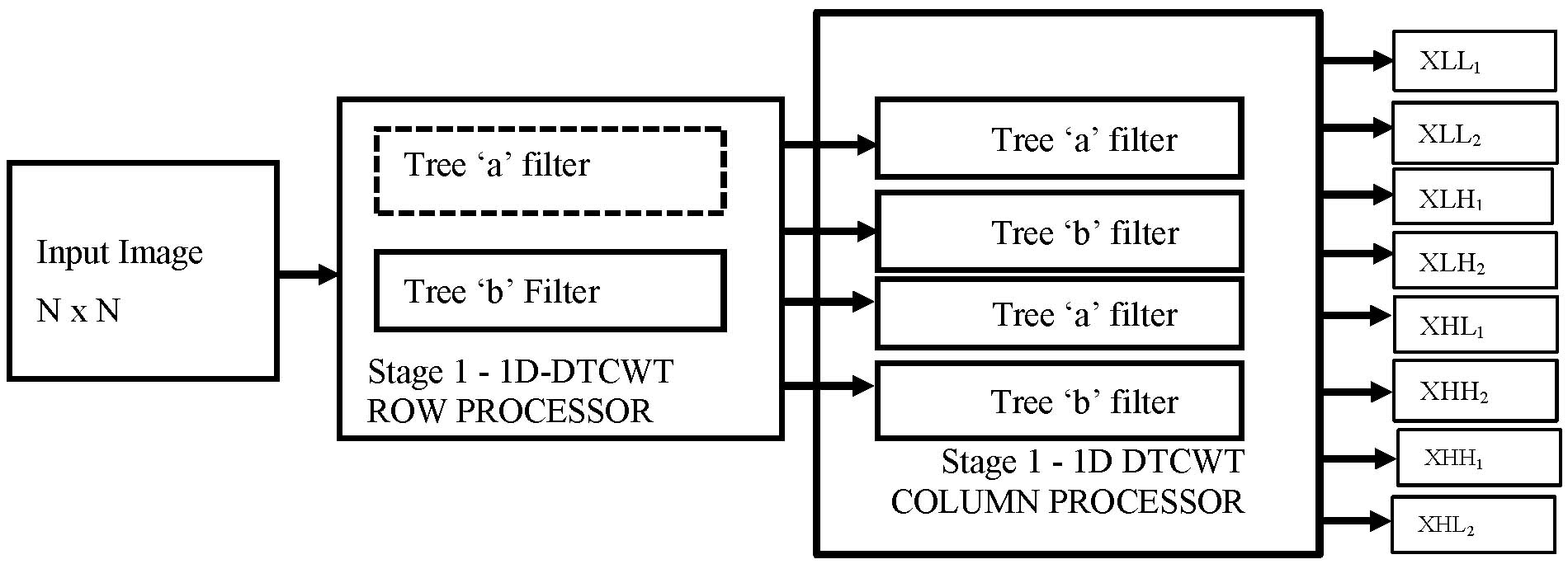##### Table 7. Implementation results of 1D DTCWT.
 Slice Logic utilization Proposed method Split DA method Number of Slice Registers 1204 2235 Number of Slice LUTs 1334 2123 Max. freq. of operation 382 MHz 395 MHz Total power dissipation (W) 0.87 1.1
##### Table 8. Implementation results of 2D DTCWT.
 FPGA utilization Proposed method Split DA method Number of Slice Registers 3564 7552 Number of Slice LUTs 3912 7682 Max. freq. of operation 312 MHz 342 MHz Total power dissipation (W) 1.01 2.04
##### Table 9. Comparison of DTCWT implementations.
 FPGA Utilization Proposed method Ref.  Ref.  Ref.  Number of Slice Register 3564 4112 7482 8799 Number of Slice LUTs 3912 4091 7224 8624 Total power (W) 1.01 1.71111 2.00207 2.192 Max. Frequency (MHz) 312 MHz 289.12 212.67 222.46

## 5. Conclusion

A DA algorithm was selected for implementing the 2D DTCWT architecture on an FPGA. The DA algorithm was modified by considering the DTCWT filters, and improved methods were designed and implemented on an FPGA. The performance metrics of DTCWT architectures developed on an FPGA were compared in terms of area, timing, and power. The high-speed DA is a recommended architecture in terms of throughput, latency, and area utilization.

### ACKNOWLEDGMENTS

This research was carried out at Reva University, Bangalore, and Cambridge Institute of Technology, Bangalore, and we acknowledge them. We also acknowledge MATLAB for providing access to the libraries.

### REFERENCES

1
R. T. Furbank, M. Tester, “Phenomics - Technologies to relieve the phenotyping bottleneck,” Trends in Plant Science, vol. 16, No. 12, pp. 635-644, 2011.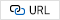2
S. Paulus, J. Behmann, A.-K. Mahlein, L. Plumer, H. Kuhlmann, “Low-cost 3D systems: Suitable tools for plant phenotyping,” Sensors, vol. 14, No. 2, 2014, pp. 3001-3018. J. L. Araus, J. E. Cairns, “Field high-throughput phenotyping: the new crop breeding frontier,”Trends in Plant Science, vol. 9, No. 1, pp. 52-61, 2013.3
L. Araus, J. E. Cairns, “Field high-throughput phenotyping: the new crop breeding frontier,”Trends in Plant Science, vol. 9, No. 1, pp. 52-61, 2013.4
Massimo Minervini, Hanno Scharr, Sotirios Tsaftaris, “The Significance of Image Compression in Plant Phenotyping Applications,” Functional Plant Biology, vol. 42, No. 10, pp. 971-988, 2015.5
Massimo Minervini, “Application-Aware Image Compression and Sensing Platform for Plant Phenotyping,” Computer Science and Engineering, IMT School for Advanced Studies, Italy, PhD Thesis, 2015.6
A. Skodras, C. Christopoulos, T. Ebrahimi, “The JPEG 2000 still image compression standard, ” IEEE Signal Processing Magazine, vol. 18, No. 5, pp. 36-58, 2001.7
N. Kingsbury, “The Dual-Tree Complex Wavelet Transform: A New Efficient Tool for Image Restoration and Enhancement,” Proceedings of 9th European Signal Processing Conference (EUSIPCO 1998), Rhodes, Greece, 8-11, pp. 1-4, Sept. 1998.8
I. W. Selesnick, R. G. Baraniuk, “The Dual-Tree Complex Wavelet Transform,” IEEE Signal Processing Magazine, vol. 22, No. 6, pp. 123-151, 2004.9
Joseph B. Boettcher, James E. Fowler, “Video Coding Using a Complex Wavelet Transform and Set Partitioning, ” IEEE Signal Processing Letters, vol. 14, pp. 633-636, 2007.10
Li Hui Fang, Miao Guo Feng, Xu Hou Jie, “Images Compression Using Dual Tree Complex Wavelet Transform, ” In Proceedings of the 2010 International Conference of Information Science and Management Engineering, IEEE Computer Society, USA, vol. 1, pp. 559-56, 2010.11
H. Naimi, A. B. H. Adamou-Mitiche, L. Mitiche, “Medical image denoising using dual tree complex thresholding wavelet transform and Wiener filter, ” Journal of King Saud University-Computer and Information Sciences, vol. 27, No. 1, pp. 40-45, 2015.12
S. S. Divakara, Sudarshan Patilkulkarni, Cyril Prasanna Raj, “High Speed Area Optimized Hybrid DA Architecture for 2D-DTCWT,” International Journal of Image and Graphics vol. 18, No. 1, 2018.13
Poornima B, Sumathi A, Cyril Prasanna Raj Premkumar, “Memory efficient high speed systolic array architecture design with multiplexed distributive arithmetic for 2D DTCWT computation on FPGA,” Journal of microelectronics, electronic components and materials, vol. 49, no. 3, pp. 119-132, 2019.14
S. S. Divakara, Sudarshan Patilkulkarni, Cyril Prasanna Raj, “High Speed Modular Systolic array based DTCWT with Parallel Processing Architecture for 2D Image Transformation on FPGA, ” International Journal of Wavelets, Multiresolution and Information Processing, vol. 15, No. 05, 1750047, 2017.## Author

Yashavantha kumar T. R. has obtained a B.E.M. tech degree from S.J.C.E, Mysore. He has 5 years of industrial experience and 10 years of teaching experience. He served in an MNC company like IBM. He joined the government sector in 2010. He worked as an HOD in the department of ECE at Government Engineering College, Karwar. He is currently working as an assistant professor in the Department of ECE at Government Engineering College, Haveri. He is pursuing his PhD at Reva University, Bangalore

S. L. Pinjare received his PhD from Indian Institute of Technology, Chennai in 1981 and has more than 40 years of experience in industry, academia and research. He worked as professor in department of ECE, NMIT, Bangalore for 15 years and has also worked in Reva University. He has worked in ITI Limited, Bangalore in VLSI department. Currently he is supervising PhD scholars in Reva University, Bangalore in the areas of signal processing, image processing, VLSI design, MEMS technology and FPGA design. He has more than 40 publications in his field of research areas and has also filed four patents. He is IEEE senior member.

Cyril Prasanna Raj P. is working as a Director at CCCIR, Cambridge Institute of Technology, Bangalore. Prior to this, he was at MS Engineering College, Bangalore, as a Research Dean for 10 years, and at MS Ramaiah University of Applied Sciences, Bangalore, as HOD in ECE Department for 12 years. He has more than 25 years of experience in teaching and research. DWT architectures over multicore platform and a novel DWT architecture for image compression have been awarded with 4 US patents. He also has 40 patents and has commercialized three products. He has more than 110 journal publications, authored 14 books, and is supervising 8 research scholars under VTU.